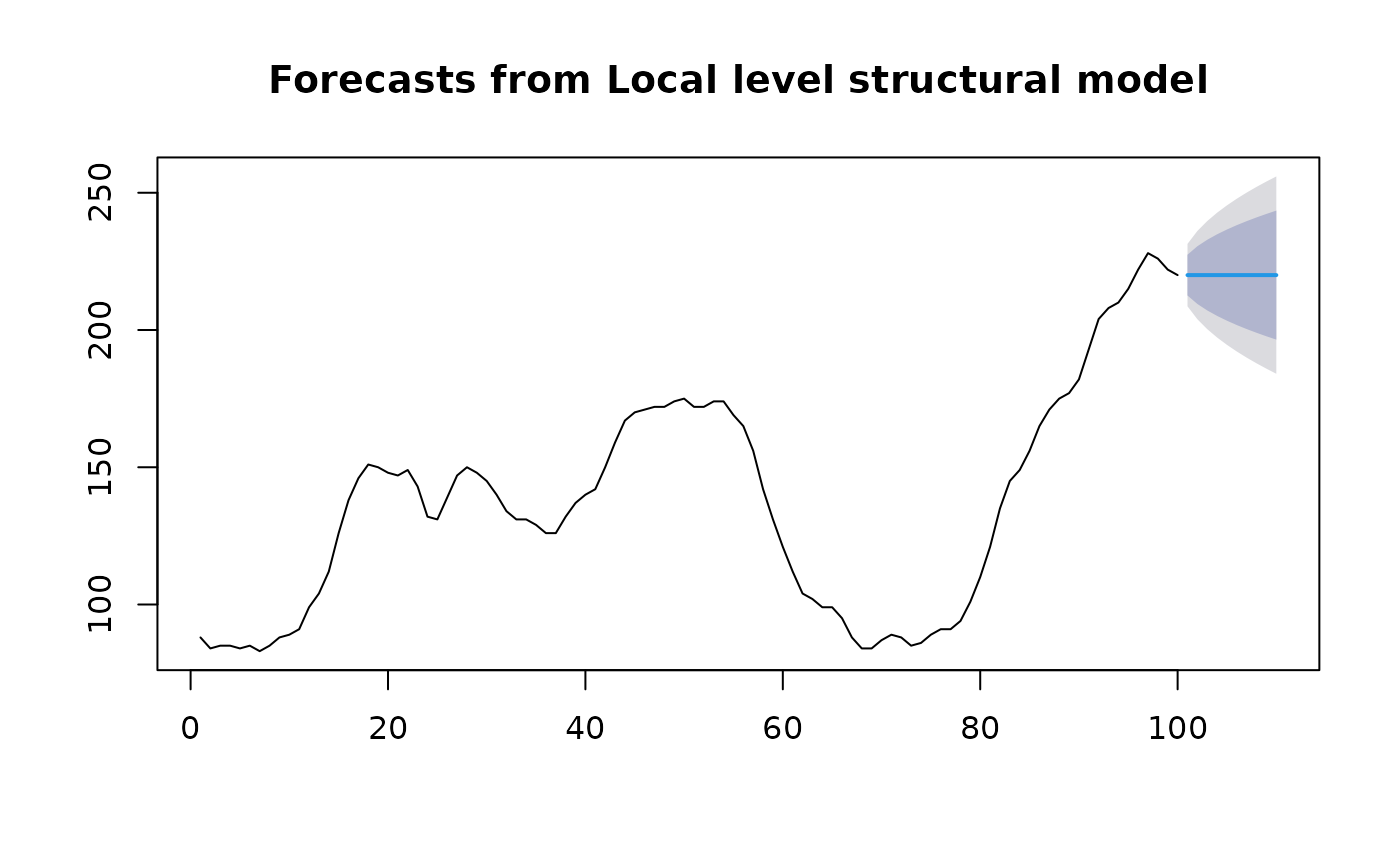Returns forecasts and other information for univariate structural time series models.

# S3 method for StructTS
forecast(
object,
h = ifelse(object$coef["epsilon"] > 1e-10, 2 * object$xtsp, 10),
level = c(80, 95),
fan = FALSE,
lambda = NULL,
...
)

## Arguments

object An object of class "StructTS". Usually the result of a call to StructTS. Number of periods for forecasting Confidence level for prediction intervals. If TRUE, level is set to seq(51,99,by=3). This is suitable for fan plots. Box-Cox transformation parameter. If lambda="auto", then a transformation is automatically selected using BoxCox.lambda. The transformation is ignored if NULL. Otherwise, data transformed before model is estimated. Use adjusted back-transformed mean for Box-Cox transformations. If transformed data is used to produce forecasts and fitted values, a regular back transformation will result in median forecasts. If biasadj is TRUE, an adjustment will be made to produce mean forecasts and fitted values. Other arguments.

## Value

An object of class "forecast".

The function summary is used to obtain and print a summary of the results, while the function plot produces a plot of the forecasts and prediction intervals.

The generic accessor functions fitted.values and residuals extract useful features of the value returned by forecast.StructTS.

An object of class "forecast" is a list containing at least the following elements:

model

A list containing information about the fitted model

method

The name of the forecasting method as a character string

mean

Point forecasts as a time series

lower

Lower limits for prediction intervals

upper

Upper limits for prediction intervals

level

The confidence values associated with the prediction intervals

x

The original time series (either object itself or the time series used to create the model stored as object).

residuals

Residuals from the fitted model. That is x minus fitted values.

fitted

Fitted values (one-step forecasts)

## Details

This function calls predict.StructTS and constructs an object of class "forecast" from the results.

StructTS.
fit <- StructTS(WWWusage,"level")